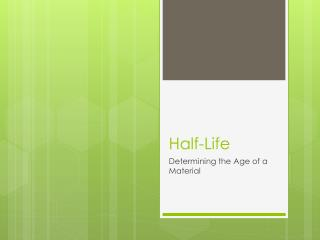DownloadDownload PresentationHalf-Life

Half-Life

Télécharger la présentationHalf-Life

- - - - - - - - - - - - - - - - - - - - - - - - - - - E N D - - - - - - - - - - - - - - - - - - - - - - - - - - -
Presentation Transcript

1. Half-Life Determining the Age of a Material

2. How to Determine the Age of Something

3. Types of Dating • Absolute (exact number) • Relative (approximate by putting in order; 1st, 2nd, 3rd, …) • Tree Rings (Dendrochronology) • Layers of Earth (Stratigraphy) • Radioisotope (Radiometric)

4. Radioisotope Dating (Radioactive Decay) • Mass can (not) be created nor destroyed • Radioactive decay means that the substance is changing (smaller by giving stuff away) • Half-life is a measurement of change (it is an average, not exact amount) • Half-life means the time it takes for ½ of something to turn into something else (not disappear)

5. The Process of Change • The percent • or fraction of • change over • time • Change • Different  • Decay •  Bigger • Smaller  Before Parent Reactant After Daughter Product

6. Common Isotope Pairs • Decay is predictable • Each parent will decay into a specific daughter • Can be 1 step or a series (many) of steps

7. Half-Life • The half-life is an approximation. A statistical average. It is how we measure the change in decay • Google map says it takes 42 minutes to drive from Vancouver to Surrey • The average Canadian home has 1.9 children and 2.25 vehicles • Canadian Life Expectancy is 80.93 years • The chance of flipping a coin 5 times and getting all heads (each time) is 1/32 • One generation is approximately 30 years

8. DEMONSTRATION Half-Life • The half-life is the rate of radioactive decay for a given isotope • It is equal to the time required for ½ of the nuclei to decay (change) • Rate  graph  curve or line (w/ slope) • x-axis = time • Y-axis = amount of material (percent or fraction) • Rate = percentage of material / time

9. Decay Curve

10. Decay Curve

11. Decay Table

12. Radioisotope Dating (Age) • Because the process of decay can be predicted or timed we can determine the age of things • If it takes 2 minutes for 500 grams of ice to turn into 250 grams at 22° Celsius • Then what can we predict:

13. We can Predict: • How much we used to have (past) • How much we will have (future) • How old is it (past) • How long will it live for (future) • How old is something (unknown) compared to something else (known) • Assume the rates (half-life) to be constant • Use the percentage or fraction of change (not the whole number) over time • ½ or 50% of the ice melts, not 2.5 grams

14. Carbon Dating • When things are alive the ratio of C-14 to C-12 are equal • But, after death C-14 decays. • Calculating the ratio will give you the age Initial: = After: >

15. Potassium Clock • When rocks are formed by lava, all gasses are freed  no Argon gas • But, after the rock is formed (hardened or cooled) then potassium decays into argon gas • Gas in rock tells age Initial: = 0 After: > 0

16. Limitations/Dangers • The age is only as accurate as the range of the isotope • Can not measure things that are older than the isotope or things that will be around longer than it. • Have to be careful with initial conditions • Therefore we have to be careful in choosing an appropriate isotope to use

17. Types of Isotopes (General) • Carbon-14 (5730 years): for life cycles (organism’s remains) • Potassium-40 (1,300 million years): for the age of the earth • Uranium-238 (4,468.3 million years): makes plutonium • Uranium-235 (703.8 million years): for reactors & Weapons

18. Types of Isotopes (Medical) • Sodium-24 (15 h): for the study of electrolytes within the body. • Chromium-51 (28 d): used to label red blood cells • Iodine-123 (13 h): thyroid function • Iodine-125 (60 d): used in cancer (prostate and brain), • Iodine-131 (8 d): organ imaging (photos)